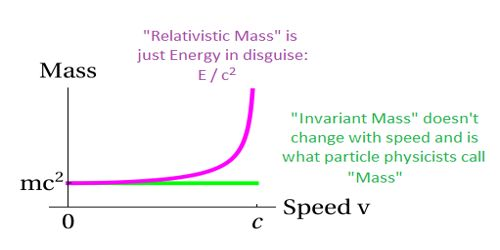# Relativity of Mass

The relativity of Mass: Like length and time, the mass of an object is dependent on motion. According to the theory of relativity mass of an object increases with a velocity of the object. It is the mass of a body in motion relative to the observer: it is equal to the rest mass multiplied by a factor that is greater than 1 and that increases as the magnitude of the velocity increases.

The term mass in special relativity usually refers to the rest mass of the object, which is the Newtonian mass as measured by an observer moving along with the object. It refers to the mass of a body which changes with the speed of the body as this speed approaches close to speed of light, it increases with velocity and tends to infinity when the velocity approaches the speed of light. The relativistic mass is a function of the rest mass and the velocity of the object.

Relativistic mass = rest mass / squared root [one minus (velocity / speed of light) squared]

The equation is: mr = m0 / sqrt (1 – v2 / c2)

Where:

mr: relativistic mass

m0: rest mass (invariant mass)

v: velocity

c: speed of light